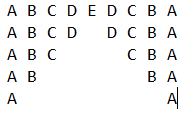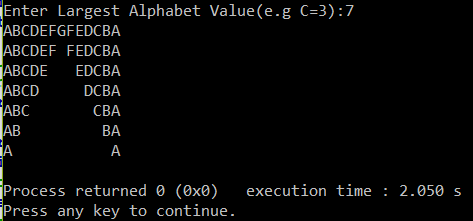Q:

# Print Reverse Triangle Bridge Pattern for Characters in C++

Reverse Triangle Bridge Pattern looks like as:To print this pattern, we can use ASCII codes of the corresponding characters to print them. Our program accepts the input of largest alphabet value in the pattern (e.g., C=3, E=5). Above Pattern shows the constant width/spacing in both the reverse triangles. By using if, else if and else statement within the nesting of for loop gives the desired result.

Program

``````#include<iostream>
using namespace std;

int main()
{
int i,j,n;
cout<<"Enter Largest Alphabet Value(e.g C=3):";
cin>>n;
for(i=0;i<n;i++)
{
for(j=65;j<64+(2*n);j++)
{
if(j>=(64+n)+i)
cout<<(char)((64+n)-(j%(64+n)));
else if(j<=(64+n)-i)
cout<<(char)j;
else
cout<<" ";
}
cout<<endl;
}

return 0;
}``````

Output